Elementary Algebra 2e

# 4.2Graph Linear Equations in Two Variables

Elementary Algebra 2e4.2 Graph Linear Equations in Two Variables

### Learning Objectives

By the end of this section, you will be able to:

• Recognize the relationship between the solutions of an equation and its graph
• Graph a linear equation by plotting points
• Graph vertical and horizontal lines

### Be Prepared 4.4

Before you get started, take this readiness quiz.

Evaluate $3x+23x+2$ when $x=−1x=−1$.
If you missed this problem, review Example 1.57.

### Be Prepared 4.5

Solve $3x+2y=123x+2y=12$ for $yy$ in general.
If you missed this problem, review Example 2.63.

### Recognize the Relationship Between the Solutions of an Equation and its Graph

In the previous section, we found several solutions to the equation $3x+2y=63x+2y=6$. They are listed in Table 4.10. So, the ordered pairs $(0,3)(0,3)$, $(2,0)(2,0)$, and $(1,32)(1,32)$ are some solutions to the equation $3x+2y=63x+2y=6$. We can plot these solutions in the rectangular coordinate system as shown in Figure 4.5.

 $3x+2y=63x+2y=6$ $xx$ $yy$ $(x,y)(x,y)$ 0 3 $(0,3)(0,3)$ 2 0 $(2,0)(2,0)$ 1 $3232$ $(1,32)(1,32)$
Table 4.10
Figure 4.5

Notice how the points line up perfectly? We connect the points with a line to get the graph of the equation $3x+2y=63x+2y=6$. See Figure 4.6. Notice the arrows on the ends of each side of the line. These arrows indicate the line continues.

Figure 4.6

Every point on the line is a solution of the equation. Also, every solution of this equation is a point on this line. Points not on the line are not solutions.

Notice that the point whose coordinates are $(−2,6)(−2,6)$ is on the line shown in Figure 4.7. If you substitute $x=−2x=−2$ and $y=6y=6$ into the equation, you find that it is a solution to the equation.

Figure 4.7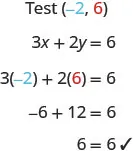So the point $(−2,6)(−2,6)$ is a solution to the equation $3x+2y=63x+2y=6$. (The phrase “the point whose coordinates are $(−2,6)(−2,6)$” is often shortened to “the point $(−2,6)(−2,6)$.”)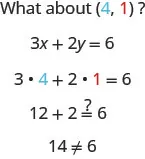So $(4,1)(4,1)$ is not a solution to the equation $3x+2y=63x+2y=6$. Therefore, the point $(4,1)(4,1)$ is not on the line. See Figure 4.6. This is an example of the saying, “A picture is worth a thousand words.” The line shows you all the solutions to the equation. Every point on the line is a solution of the equation. And, every solution of this equation is on this line. This line is called the graph of the equation $3x+2y=63x+2y=6$.

### Graph of a Linear Equation

The graph of a linear equation $Ax+By=CAx+By=C$ is a line.

• Every point on the line is a solution of the equation.
• Every solution of this equation is a point on this line.

### Example 4.10

The graph of $y=2x−3y=2x−3$ is shown.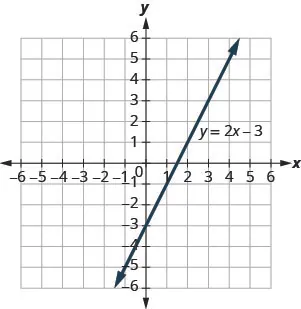For each ordered pair, decide:

Is the ordered pair a solution to the equation?
Is the point on the line?

A $(0,−3)(0,−3)$ B $(3,3)(3,3)$ C $(2,−3)(2,−3)$ D $(−1,−5)(−1,−5)$

### Try It 4.19

Use the graph of $y=3x−1y=3x−1$ to decide whether each ordered pair is:

• a solution to the equation.
• on the line.

$(0,−1)(0,−1)$ $(2,5)(2,5)$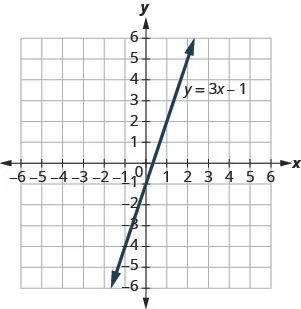### Try It 4.20

Use graph of $y=3x−1y=3x−1$ to decide whether each ordered pair is:

• a solution to the equation
• on the line

$(3,−1)(3,−1)$ $(−1,−4)(−1,−4)$### Graph a Linear Equation by Plotting Points

There are several methods that can be used to graph a linear equation. The method we used to graph $3x+2y=63x+2y=6$ is called plotting points, or the Point–Plotting Method.

### Example 4.11

#### How To Graph an Equation By Plotting Points

Graph the equation $y=2x+1y=2x+1$ by plotting points.

### Try It 4.21

Graph the equation by plotting points: $y=2x−3y=2x−3$.

### Try It 4.22

Graph the equation by plotting points: $y=−2x+4y=−2x+4$.

The steps to take when graphing a linear equation by plotting points are summarized below.

### How To

#### Graph a linear equation by plotting points.

1. Step 1. Find three points whose coordinates are solutions to the equation. Organize them in a table.
2. Step 2. Plot the points in a rectangular coordinate system. Check that the points line up. If they do not, carefully check your work.
3. Step 3. Draw the line through the three points. Extend the line to fill the grid and put arrows on both ends of the line.

It is true that it only takes two points to determine a line, but it is a good habit to use three points. If you only plot two points and one of them is incorrect, you can still draw a line but it will not represent the solutions to the equation. It will be the wrong line.

If you use three points, and one is incorrect, the points will not line up. This tells you something is wrong and you need to check your work. Look at the difference between part (a) and part (b) in Figure 4.8.

Figure 4.8

Let’s do another example. This time, we’ll show the last two steps all on one grid.

### Example 4.12

Graph the equation $y=−3xy=−3x$.

### Try It 4.23

Graph the equation by plotting points: $y=−4xy=−4x$.

### Try It 4.24

Graph the equation by plotting points: $y=xy=x$.

When an equation includes a fraction as the coefficient of $xx$, we can still substitute any numbers for $xx$. But the math is easier if we make ‘good’ choices for the values of $xx$. This way we will avoid fraction answers, which are hard to graph precisely.

### Example 4.13

Graph the equation $y=12x+3y=12x+3$.

### Try It 4.25

Graph the equation $y=13x−1y=13x−1$.

### Try It 4.26

Graph the equation $y=14x+2y=14x+2$.

So far, all the equations we graphed had $yy$ given in terms of $xx$. Now we’ll graph an equation with $xx$ and $yy$ on the same side. Let’s see what happens in the equation $2x+y=32x+y=3$. If $y=0y=0$ what is the value of $xx$?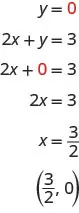This point has a fraction for the x- coordinate and, while we could graph this point, it is hard to be precise graphing fractions. Remember in the example $y=12x+3y=12x+3$, we carefully chose values for $xx$ so as not to graph fractions at all. If we solve the equation $2x+y=32x+y=3$ for $yy$, it will be easier to find three solutions to the equation.

$2x+y=3y=−2x+32x+y=3y=−2x+3$

The solutions for $x=0x=0$, $x=1x=1$, and $x=−1x=−1$ are shown in the Table 4.13. The graph is shown in Figure 4.9.

 $2x+y=32x+y=3$ $xx$ $yy$ $(x,y)(x,y)$ 0 3 $(0,3)(0,3)$ 1 1 $(1,1)(1,1)$ $−1−1$ 5 $(−1,5)(−1,5)$
Table 4.13
Figure 4.9

Can you locate the point $(32,0)(32,0)$, which we found by letting $y=0y=0$, on the line?

### Example 4.14

Graph the equation $3x+y=−13x+y=−1$.

### Try It 4.27

Graph the equation $2x+y=22x+y=2$.

### Try It 4.28

Graph the equation $4x+y=−34x+y=−3$.

If you can choose any three points to graph a line, how will you know if your graph matches the one shown in the answers in the book? If the points where the graphs cross the x- and y-axis are the same, the graphs match!

The equation in Example 4.14 was written in standard form, with both $xx$ and $yy$ on the same side. We solved that equation for $yy$ in just one step. But for other equations in standard form it is not that easy to solve for $yy$, so we will leave them in standard form. We can still find a first point to plot by letting $x=0x=0$ and solving for $yy$. We can plot a second point by letting $y=0y=0$ and then solving for $xx$. Then we will plot a third point by using some other value for $xx$ or $yy$.

### Example 4.15

Graph the equation $2x−3y=62x−3y=6$.

### Try It 4.29

Graph the equation $4x+2y=84x+2y=8$.

### Try It 4.30

Graph the equation $2x−4y=82x−4y=8$.

### Graph Vertical and Horizontal Lines

Can we graph an equation with only one variable? Just $xx$ and no $yy$, or just $yy$ without an $xx$? How will we make a table of values to get the points to plot?

Let’s consider the equation $x=−3x=−3$. This equation has only one variable, $xx$. The equation says that $xx$ is always equal to $−3−3$, so its value does not depend on $yy$. No matter what $yy$ is, the value of $xx$ is always $−3−3$.

So to make a table of values, write $−3−3$ in for all the $xx$ values. Then choose any values for $yy$. Since $xx$ does not depend on $yy$, you can choose any numbers you like. But to fit the points on our coordinate graph, we’ll use 1, 2, and 3 for the y-coordinates. See Table 4.18.

 $x=−3x=−3$ $xx$ $yy$ $(x,y)(x,y)$ $−3−3$ 1 $(−3,1)(−3,1)$ $−3−3$ 2 $(−3,2)(−3,2)$ $−3−3$ 3 $(−3,3)(−3,3)$
Table 4.18

Plot the points from Table 4.18 and connect them with a straight line. Notice in Figure 4.12 that we have graphed a vertical line.

Figure 4.12

### Vertical Line

A vertical line is the graph of an equation of the form $x=ax=a$.

The line passes through the x-axis at $(a,0)(a,0)$.

### Example 4.16

Graph the equation $x=2x=2$.

### Try It 4.31

Graph the equation $x=5x=5$.

### Try It 4.32

Graph the equation $x=−2x=−2$.

What if the equation has $yy$ but no $xx$? Let’s graph the equation $y=4y=4$. This time the y- value is a constant, so in this equation, $yy$ does not depend on $xx$. Fill in 4 for all the $yy$’s in Table 4.20 and then choose any values for $xx$. We’ll use 0, 2, and 4 for the x-coordinates.

 $y=4y=4$ $xx$ $yy$ $(x,y)(x,y)$ 0 4 $(0,4)(0,4)$ 2 4 $(2,4)(2,4)$ 4 4 $(4,4)(4,4)$
Table 4.20

The graph is a horizontal line passing through the y-axis at 4. See Figure 4.14.

Figure 4.14

### Horizontal Line

A horizontal line is the graph of an equation of the form $y=by=b$.

The line passes through the y-axis at $(0,b)(0,b)$.

### Example 4.17

Graph the equation $y=−1.y=−1.$

### Try It 4.33

Graph the equation $y=−4y=−4$.

### Try It 4.34

Graph the equation $y=3y=3$.

The equations for vertical and horizontal lines look very similar to equations like $y=4x.y=4x.$ What is the difference between the equations $y=4xy=4x$ and $y=4y=4$?

The equation $y=4xy=4x$ has both $xx$ and $yy$. The value of $yy$ depends on the value of $xx$. The y-coordinate changes according to the value of $xx$. The equation $y=4y=4$ has only one variable. The value of $yy$ is constant. The y-coordinate is always 4. It does not depend on the value of $xx$. See Table 4.22.

 $y=4xy=4x$ $y=4y=4$ $xx$ $yy$ $(x,y)(x,y)$ $xx$ $yy$ $(x,y)(x,y)$ 0 0 $(0,0)(0,0)$ 0 4 $(0,4)(0,4)$ 1 4 $(1,4)(1,4)$ 1 4 $(1,4)(1,4)$ 2 8 $(2,8)(2,8)$ 2 4 $(2,4)(2,4)$
Table 4.22
Figure 4.16

Notice, in Figure 4.16, the equation $y=4xy=4x$ gives a slanted line, while $y=4y=4$ gives a horizontal line.

### Example 4.18

Graph $y=−3xy=−3x$ and $y=−3y=−3$ in the same rectangular coordinate system.

### Try It 4.35

Graph $y=−4xy=−4x$ and $y=−4y=−4$ in the same rectangular coordinate system.

### Try It 4.36

Graph $y=3y=3$ and $y=3xy=3x$ in the same rectangular coordinate system.

### Section 4.2 Exercises

#### Practice Makes Perfect

Recognize the Relationship Between the Solutions of an Equation and its Graph

In the following exercises, for each ordered pair, decide:

Is the ordered pair a solution to the equation?  Is the point on the line?

55.

$y = x + 2 y = x + 2$

1. $(0,2)(0,2)$
2. $(1,2)(1,2)$
3. $(−1,1)(−1,1)$
4. $(−3,−1)(−3,−1)$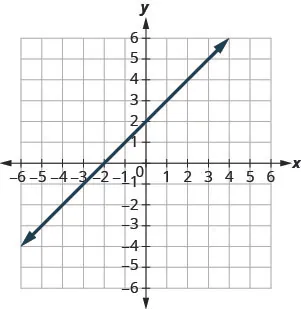56.

$y = x − 4 y = x − 4$

1. $(0,−4)(0,−4)$
2. $(3,−1)(3,−1)$
3. $(2,2)(2,2)$
4. $(1,−5)(1,−5)$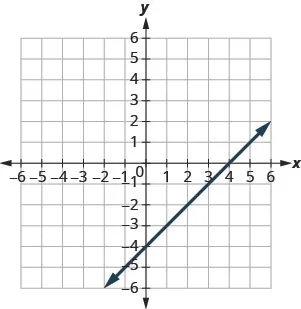57.

$y = 1 2 x − 3 y = 1 2 x − 3$

1. $(0,−3)(0,−3)$
2. $(2,−2)(2,−2)$
3. $(−2,−4)(−2,−4)$
4. $(4,1)(4,1)$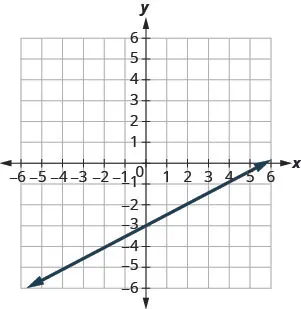58.

$y = 1 3 x + 2 y = 1 3 x + 2$

1. $(0,2)(0,2)$
2. $(3,3)(3,3)$
3. $(−3,2)(−3,2)$
4. $(−6,0)(−6,0)$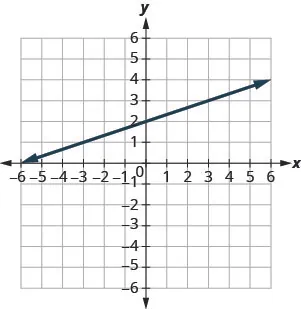Graph a Linear Equation by Plotting Points

In the following exercises, graph by plotting points.

59.

$y = 3 x − 1 y = 3 x − 1$

60.

$y = 2 x + 3 y = 2 x + 3$

61.

$y = −2 x + 2 y = −2 x + 2$

62.

$y = −3 x + 1 y = −3 x + 1$

63.

$y = x + 2 y = x + 2$

64.

$y = x − 3 y = x − 3$

65.

$y = − x − 3 y = − x − 3$

66.

$y = − x − 2 y = − x − 2$

67.

$y = 2 x y = 2 x$

68.

$y = 3 x y = 3 x$

69.

$y = −4 x y = −4 x$

70.

$y = −2 x y = −2 x$

71.

$y = 1 2 x + 2 y = 1 2 x + 2$

72.

$y = 1 3 x − 1 y = 1 3 x − 1$

73.

$y = 4 3 x − 5 y = 4 3 x − 5$

74.

$y = 3 2 x − 3 y = 3 2 x − 3$

75.

$y = − 2 5 x + 1 y = − 2 5 x + 1$

76.

$y = − 4 5 x − 1 y = − 4 5 x − 1$

77.

$y = − 3 2 x + 2 y = − 3 2 x + 2$

78.

$y = − 5 3 x + 4 y = − 5 3 x + 4$

79.

$x + y = 6 x + y = 6$

80.

$x + y = 4 x + y = 4$

81.

$x + y = −3 x + y = −3$

82.

$x + y = −2 x + y = −2$

83.

$x − y = 2 x − y = 2$

84.

$x − y = 1 x − y = 1$

85.

$x − y = −1 x − y = −1$

86.

$x − y = −3 x − y = −3$

87.

$3 x + y = 7 3 x + y = 7$

88.

$5 x + y = 6 5 x + y = 6$

89.

$2 x + y = −3 2 x + y = −3$

90.

$4 x + y = −5 4 x + y = −5$

91.

$1 3 x + y = 2 1 3 x + y = 2$

92.

$1 2 x + y = 3 1 2 x + y = 3$

93.

$2 5 x − y = 4 2 5 x − y = 4$

94.

$3 4 x − y = 6 3 4 x − y = 6$

95.

$2 x + 3 y = 12 2 x + 3 y = 12$

96.

$4 x + 2 y = 12 4 x + 2 y = 12$

97.

$3 x − 4 y = 12 3 x − 4 y = 12$

98.

$2 x − 5 y = 10 2 x − 5 y = 10$

99.

$x − 6 y = 3 x − 6 y = 3$

100.

$x − 4 y = 2 x − 4 y = 2$

101.

$5 x + 2 y = 4 5 x + 2 y = 4$

102.

$3 x + 5 y = 5 3 x + 5 y = 5$

Graph Vertical and Horizontal Lines

In the following exercises, graph each equation.

103.

$x = 4 x = 4$

104.

$x = 3 x = 3$

105.

$x = −2 x = −2$

106.

$x = −5 x = −5$

107.

$y = 3 y = 3$

108.

$y = 1 y = 1$

109.

$y = −5 y = −5$

110.

$y = −2 y = −2$

111.

$x = 7 3 x = 7 3$

112.

$x = 5 4 x = 5 4$

113.

$y = − 15 4 y = − 15 4$

114.

$y = − 5 3 y = − 5 3$

In the following exercises, graph each pair of equations in the same rectangular coordinate system.

115.

$y=2xy=2x$ and $y=2y=2$

116.

$y=5xy=5x$ and $y=5y=5$

117.

$y=−12xy=−12x$ and $y=−12y=−12$

118.

$y=−13xy=−13x$ and $y=−13y=−13$

#### Mixed Practice

In the following exercises, graph each equation.

119.

$y = 4 x y = 4 x$

120.

$y = 2 x y = 2 x$

121.

$y = − 1 2 x + 3 y = − 1 2 x + 3$

122.

$y = 1 4 x − 2 y = 1 4 x − 2$

123.

$y = − x y = − x$

124.

$y = x y = x$

125.

$x − y = 3 x − y = 3$

126.

$x + y = −5 x + y = −5$

127.

$4 x + y = 2 4 x + y = 2$

128.

$2 x + y = 6 2 x + y = 6$

129.

$y = −1 y = −1$

130.

$y = 5 y = 5$

131.

$2 x + 6 y = 12 2 x + 6 y = 12$

132.

$5 x + 2 y = 10 5 x + 2 y = 10$

133.

$x = 3 x = 3$

134.

$x = −4 x = −4$

#### Everyday Math

135.

Motor home cost. The Robinsons rented a motor home for one week to go on vacation. It cost them $594 plus$0.32 per mile to rent the motor home, so the linear equation $y=594+0.32xy=594+0.32x$ gives the cost, $yy$, for driving $xx$ miles. Calculate the rental cost for driving 400, 800, and 1200 miles, and then graph the line.

136.

Weekly earnings. At the art gallery where he works, Salvador gets paid $200 per week plus 15% of the sales he makes, so the equation $y=200+0.15xy=200+0.15x$ gives the amount, $yy$, he earns for selling $xx$ dollars of artwork. Calculate the amount Salvador earns for selling$900, $1600, and$2000, and then graph the line.

#### Writing Exercises

137.

Explain how you would choose three x- values to make a table to graph the line $y=15x−2y=15x−2$.

138.

What is the difference between the equations of a vertical and a horizontal line?

#### Self Check

After completing the exercises, use this checklist to evaluate your mastery of the objectives of this section.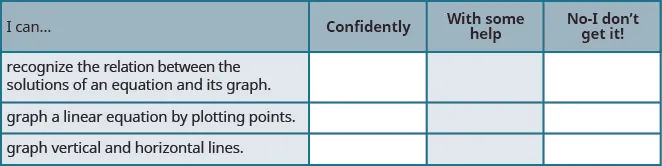After reviewing this checklist, what will you do to become confident for all goals?

Order a print copy

As an Amazon Associate we earn from qualifying purchases.